Дана: 0

Барлыгы: 0,00

0

# Net of a cube (exercises)

### Net of a cube (exercises)

Not all nets consisting of 6 congruent squares are foldable into cubes.

Математика

Этикеткалар

net of a cube, куб, net, regular solids, solids, геометрия, exercise, математика

Байланысты экстралар

### Көріністер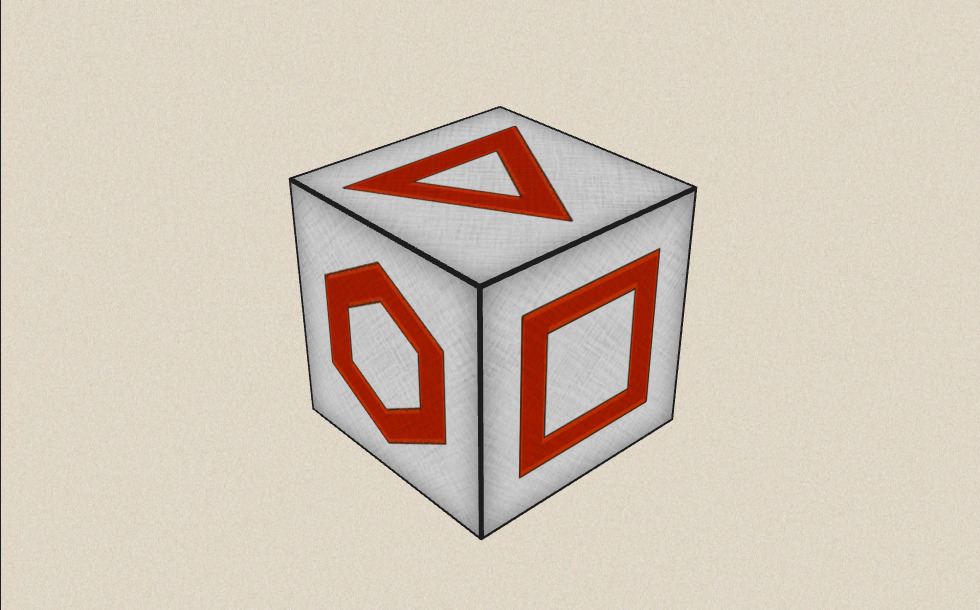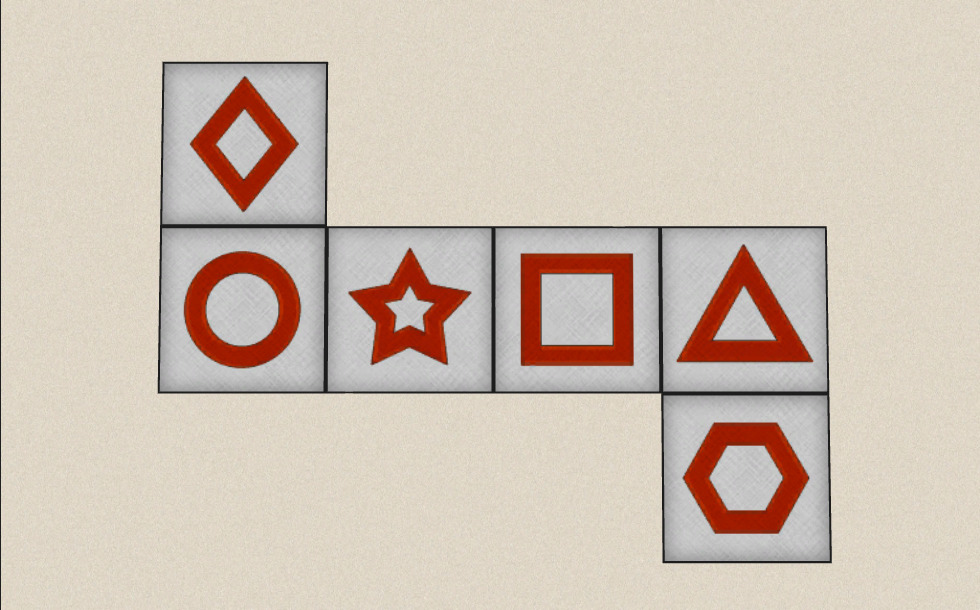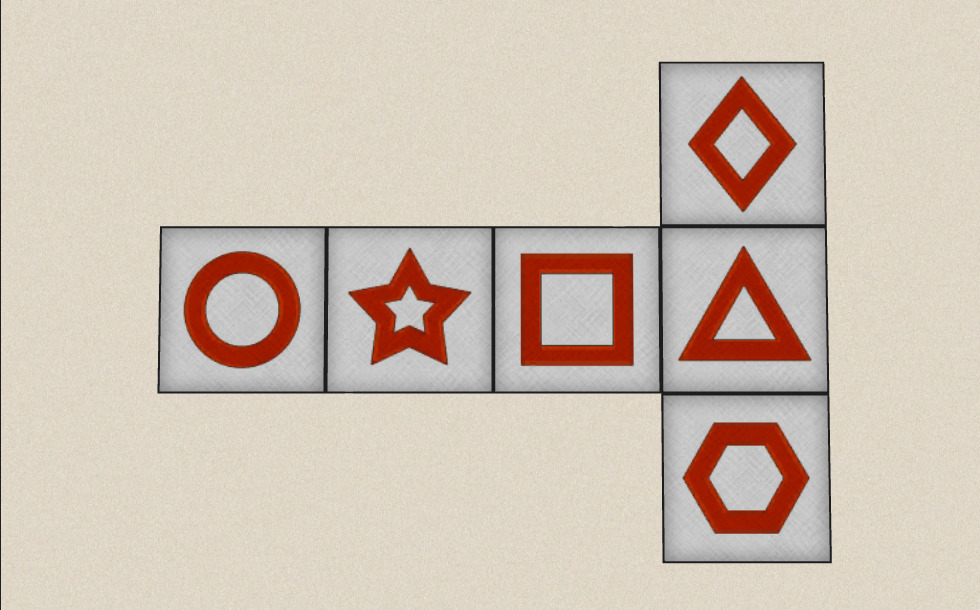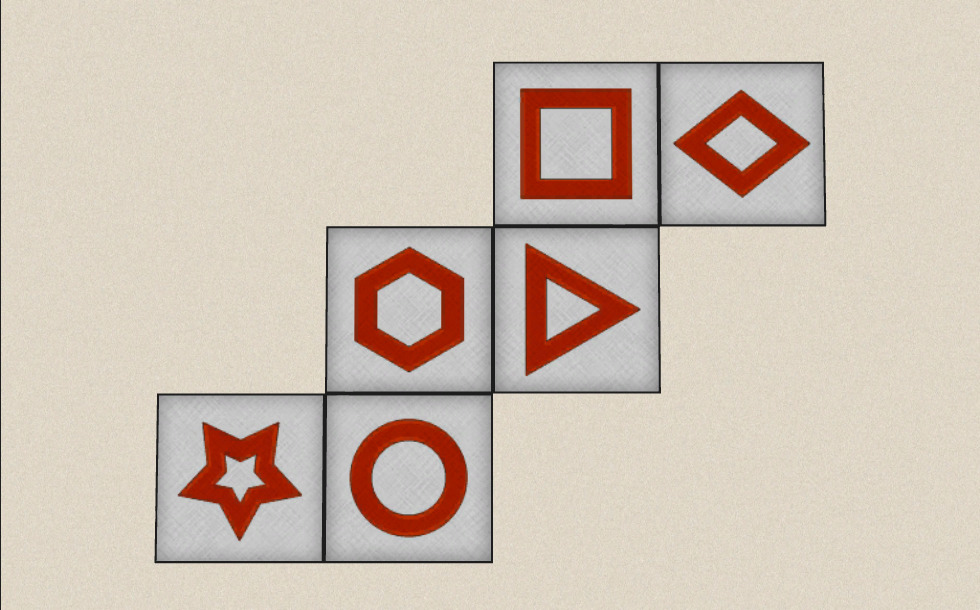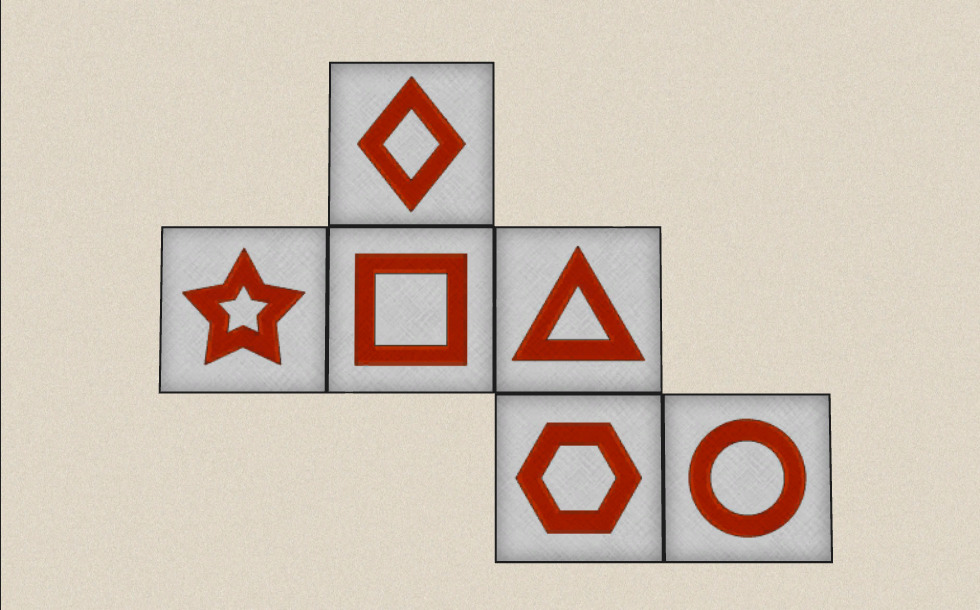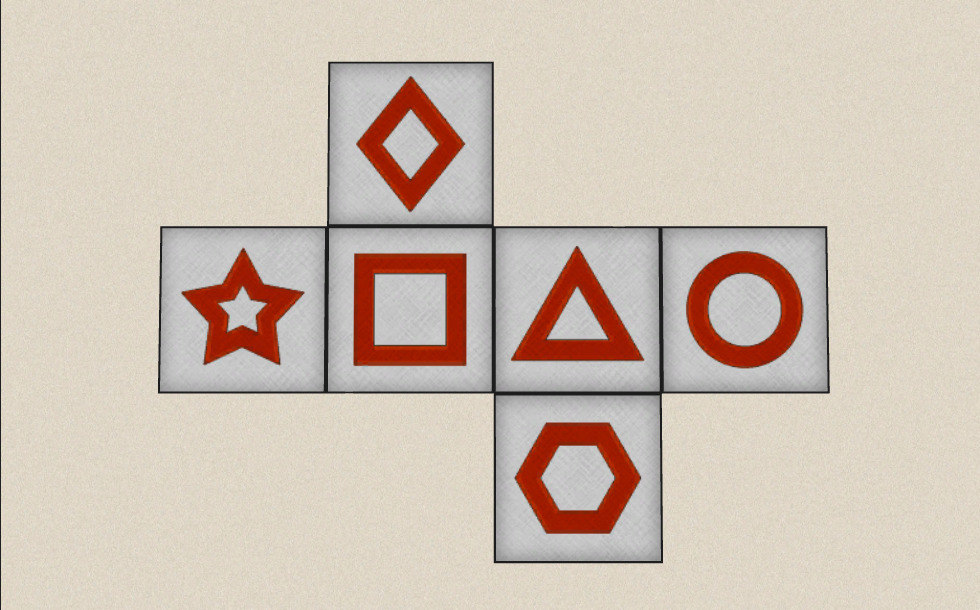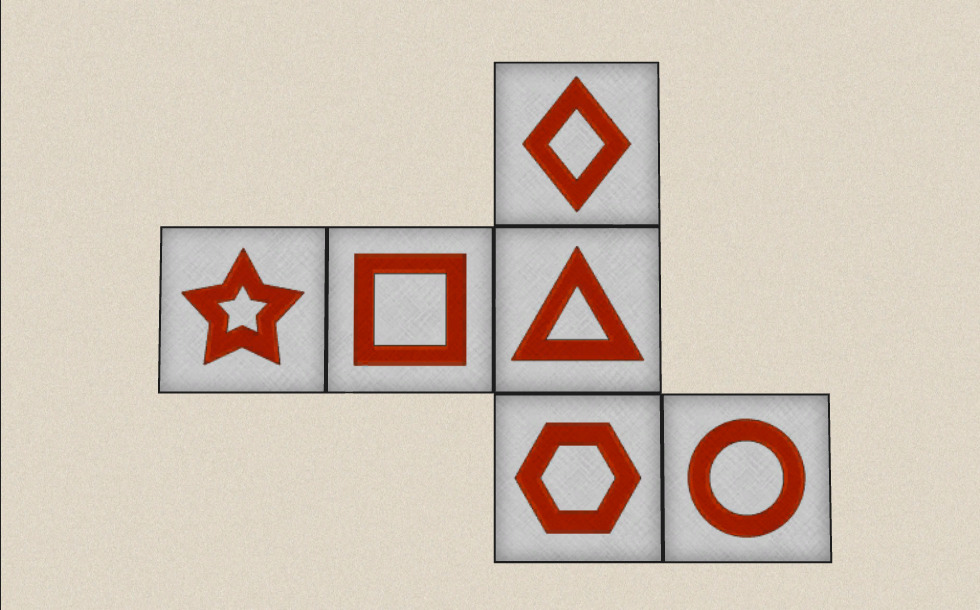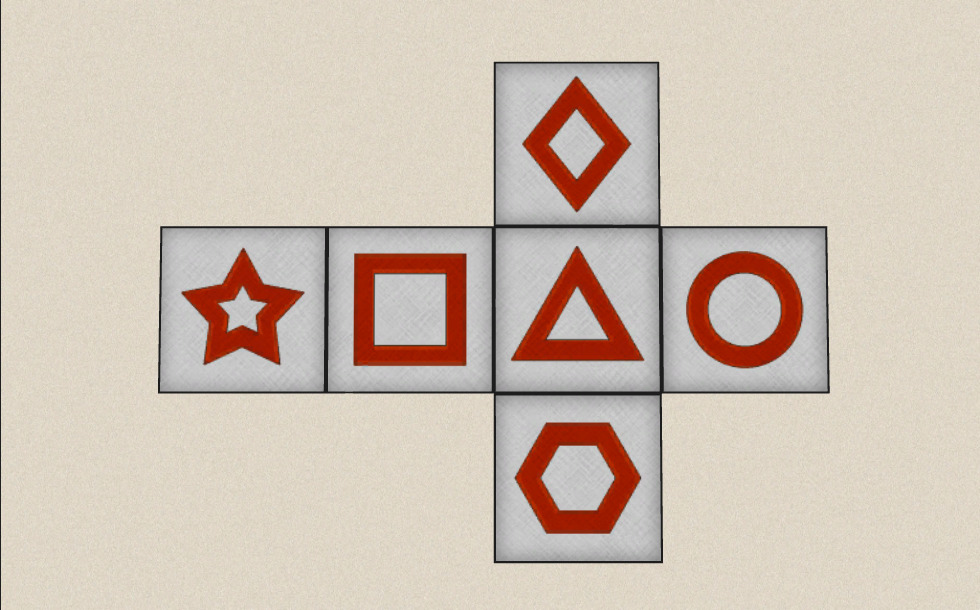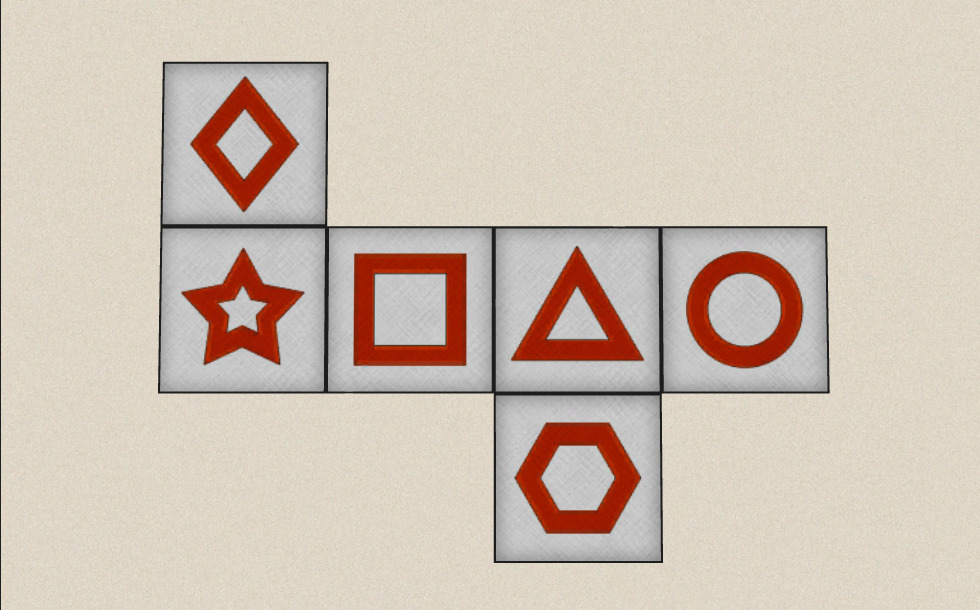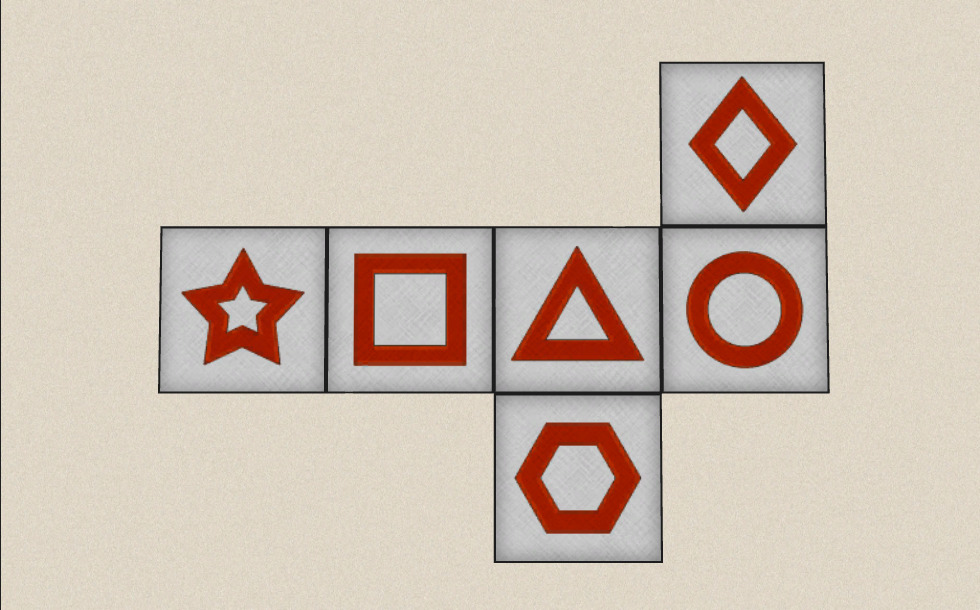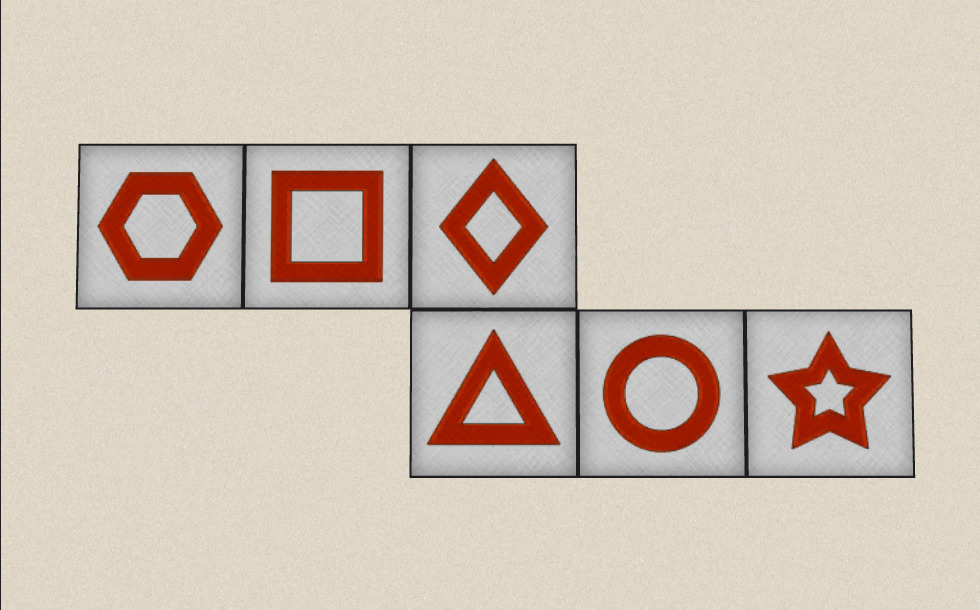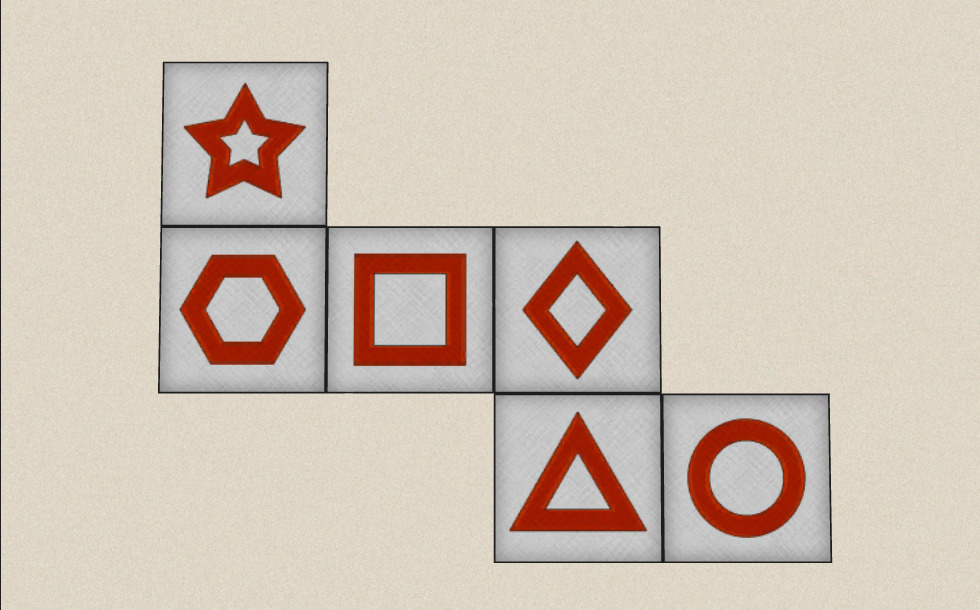### Байланысты экстралар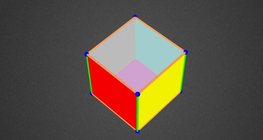#### Colouring a cube

Colouring the vertices, edges and faces of a given cube according to the criteria...#### Cube

This animation demonstrates the components (vertices, edges, diagonals and faces) of the...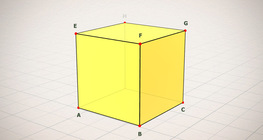#### Cube (exercises)

Edges, diagonals and faces of a cube can be identified by its vertices.#### Cube of cubes

An exercise about the regular hexahedron built from unit cubes to help deepen your...#### Cube puzzle

Building cubes shown in several views from the available unit cubes aids spatial vision...#### Cube sections (exercise)

Examining solids formed by the intersection of a cube and a plane.#### Қысқаша көбейтудің формулалары

Анимацияда қысқаша көбейту формулаларының ұқсас қосылғыштары көрсетіледі.#### Жазық фигуралардың периметрі мен ауданын, беттің ауданын және көлемін есептеу

Анимацияның көмегімен жазық фигуралардың периметрі мен ауданын есептеу формуласымен,...#### Building shapes (multi colour)

Build 3D shapes from unit cubes with the help of several views.#### Building shapes (one colour)

Build 3D shapes from unit cubes with the help of several views.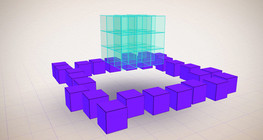#### Constructing shapes (3D)

Building 3D shapes into a given cube mesh with the help of several views.#### Cuboid

A cuboid is a polyhedron with six rectangular faces.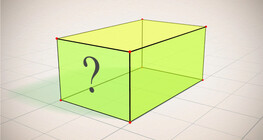#### Cuboid (exercises)

Edges, diagonals and faces of a cuboid can be identified by its vertices.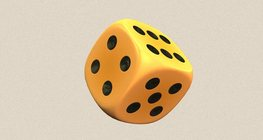#### Dice

Regular dice can be used for solving statistical and probability exercises.#### Grouping of cuboids

This animation demonstrates various types of cuboids through everyday objects.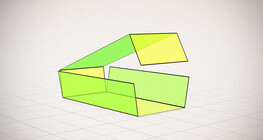#### Nets of a cuboid (exercises)

This animation demonstrates the different nets of a cuboid and includes a game.#### Platonic solids

This animation demonstrates the five regular three-dimensional (or Platonic) solids, the...#### Shape games

Find views and nets of three-dimensional shapes.#### Volume and surface area (exercise)

An exercise about the volume and surface area of solids generated from a ´base cube´.